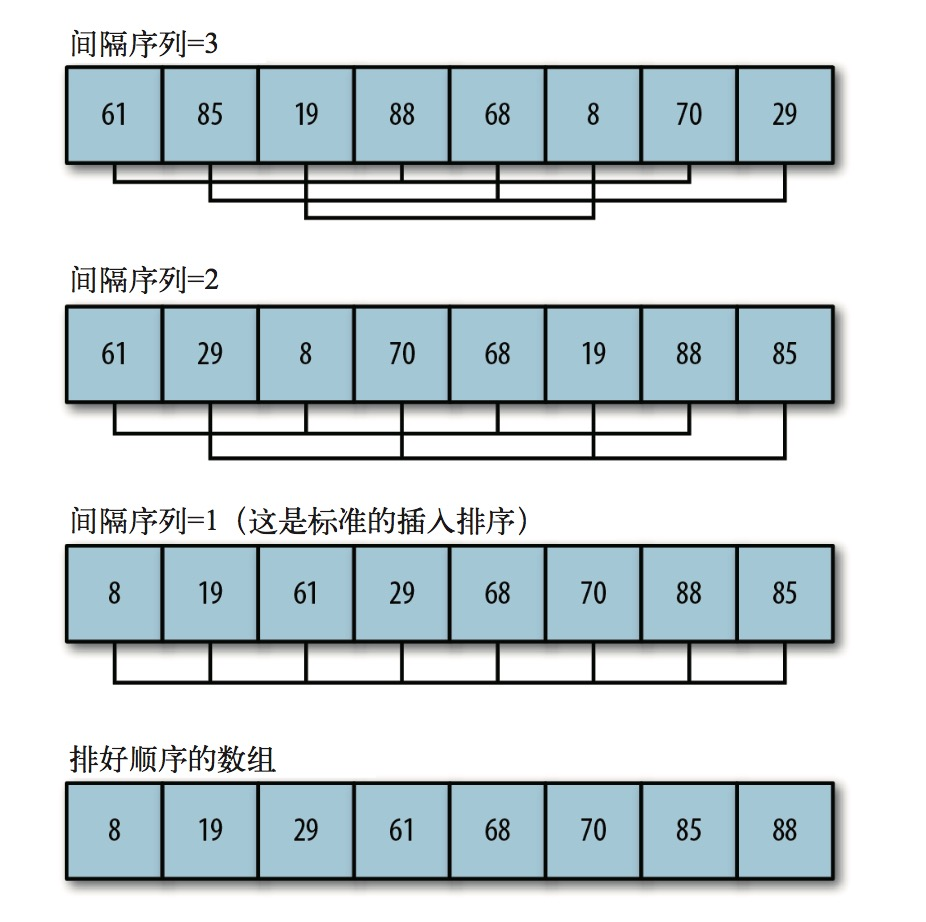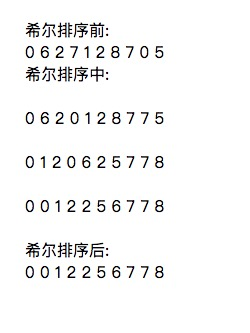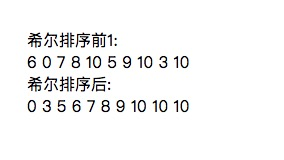# 高级排序— 希尔排序

### 希尔排序

``````//希尔排序
function shellsort() {
for (var g = 0; g < this.gaps.length; ++g) {
for (var i = this.gaps[g]; i < this.dataStore.length; ++i) {
var temp = this.dataStore[i];
for (var j = i; j >= this.gaps[g] &&
this.dataStore[j-this.gaps[g]] > temp;
j -= this.gaps[g]) {
this.dataStore[j] = this.dataStore[j - this.gaps[g]];
}
this.dataStore[j] = temp;
}
document.write('<br />');
document.write(this.toString());
document.write('<br />');
}
}

function setGaps(arr) {
this.gaps = arr;
}

``````

``````this.gaps = [5,3,1];
````````````    var nums = new CArray(100);
nums.setData();
document.write("希尔排序前:<br />");
document.write(nums.toString());
document.write("<br />希尔排序中:<br />");
nums.shellsort();
document.write("<br />希尔排序后:<br />");
document.write(nums.toString());
``````### 动态计算间隔序列的希尔排序

《算法(第4版)》(人民邮电出版社)的合著者Robert Sedgewick定义了一个shellsort() 函数，在这个函数中可以通过一个公式来对希尔排序用到的间隔序列进行动态计算。 Sedgewick 的算法是通过下面的代码片段来决定初始间隔值的:

``````    var N = this.dataStore.length;
var h = 1;
while (h < N/3) {
h = 3 * h + 1;
}
``````

h = (h-1)/3;

``````function shellsort1() {
var N = this.dataStore.length;
var h = 1;
while (h < N/3) {
h = 3 * h + 1;
}
while (h >= 1) {
for (var i = h; i < N; i++) {
for (var j = i; j >= h && this.dataStore[j] < this.dataStore[j-h];
j -= h) {
swap(this.dataStore, j, j-h);
}
}
h = (h-1)/3;
}
}
``````### 比较 shellsort() 算法

``````    var nums = new CArray(100000);
nums.setData();
var start = new Date().getTime();
nums.shellsort();
var stop = new Date().getTime();
var elapsed = stop - start;
document.write(" 硬编码间隔序列的希尔排序消耗的时间为:" + elapsed + " 毫秒。");
nums.clear();

nums.setData();
start = new Date().getTime();
nums.shellsort1();
stop = new Date().getTime();
document.write(" 动态间隔序列的希尔排序消耗的时间为:" + elapsed + " 毫秒。");
``````